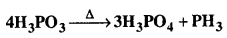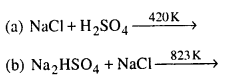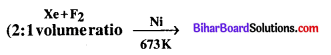Bihar Board 12th Chemistry Objective Questions and Answers

## Bihar Board 12th Chemistry Objective Answers Chapter 7 The p-Block Elements

Question 1.
Nitrogen shows different oxidation states ranging from
(a) -3 to +5
(b) -5 to + 5
(c) 0 to -5
(d) -3 to +3
(a) -3 to +5

Question 2.
The oxidation state of nitrogen is highest in
(a) N3H
(b) NH3
(c) NH2OH
(d) N2H4
(a) N3HQuestion 3.
Nitrogen forms stable N2 molecule but phosphorus is converted to P4 from P2 because
(a) pπ – pπ bonding is strong in phosphorus
(b) pπ – pπ bonding is weak in phosphorus
(c) triple bond is present in phosphorus
(d) single P – P bond is weaker than N – N bond
(b) pπ – pπ bonding is weak in phosphorus

Question 4.
The decreasing order of boiling points of the following hydrides is …………..
(a) H2O > SbH3 > AsH3 > PH3 > NH3
(b) H2O > NH3 > SbH3 > AsH3 > PH3
(c) H2O > SbH3 > NH3 > AsH3 > PH3
(d) H2O > PH3 > AsH3 > SbH3 > NH3
(c) H2O>SbH3>NH3>AsH3>PH3

Question 5.
Nitrogen can form only one chloride with chlorine which is NCl3 where as P can form PCl3 and PCl5. This is
(a) due to absence of d – orbitals in nitrogen
(b) due to difference in size of N and P
(c) due to higher reactivity of P towards Cl than N
(d) due to presence of multiple bonding in nitrogen
(a) due to absence of d – orbitals in nitrogen

Question 6.
On heating a mixture of NH4Cl and KNO2, we get
(a) NH4NO3
(b) KNH4(NO3)2
(c) N2
(d) NO
(c) N2

Question 7.
Nitrogen is relatively inactive element because
(a) its atoms has a stable electronic configuration
(b) it has low atomic radius
(c) its electronegativity is fairly high
(d) dissociation energy of its molecule is fairly high
(d) dissociation energy of its molecule is fairly highQuestion 8.
Nitrogen combines with metals to form
(a) nitrites
(b) nitrates
(c) nitrosyl chloride
(d) nitrides
(d) nitrides

Question 9.
Nitrogen is used to fill electric bulbs because
(a) it is lighter than air
(b) it makes the bulb to glow
(c) it does not support combustion
(d) it is non-toxic
(c) it does not support combustion

Question 10.
Which of the following compounds will not give ammount on heathing ?
(a) (NH4)2SO4
(b) (NH4)2CO3
(c) NH4NO2
(d) NH4Cl
(c) NH4NO2

Question 11.
Which of the following factors would favour the formation of ammonia ?
(a) High pressure
(b) Low temperatue
(c) High volume
(d) Low pressure
(a) High pressureQuestion 12.
Ammonia is a Lewis base. It forms complexes with cations. Which one of the following cations does not form complex with ammonia ?
(a) Ag+
(b) Cu2+
(c) Cd2+
(d) Pb2+
(d) Pb2+

Question 13.
Which oxide of nitrogen is obtained on heating ammonium nitrate at 250°C ?
(a) Nitric oxide
(b) Nitrous oxide
(c) Nitrogen dioxide
(d) Dinitrogen  tetraoxide
(b) Nitrous oxide

Question 14.
Which of the following oxides is anhydride of nitrous acid ?
(a) N2O3
(b) NO2
(c) NO
(d) N2O4
(a) N2O3

Question 15.
Atomicity of phosphorus is
(a) one
(b) two
(c) three
(d) four
(d) fourQuestion 16.
The structure of white phosphorus is ‘
(a) square planar
(b) pyramidal
(c) tetrahedral
(d) trigonal planar
(c) tetrahedral

Question 17.
Each of the following is true for white and red phosphorus except that they
(a) are both soluble in CS2
(b) can be oxidized by heating in air
(c) consist of the same kind of atoms
(d) can be converted into one another
(a) are both soluble in CS2

Question 18.
Phosphine is prepared by the action of
(a) P and H2SO4
(b) P and NaOH
(c) P and H2S
(d) P and HNO3
(b) P and NaOHQuestion 19.
Holme’s signal uses chemical compound
(a) calcium carbide
(b) calcium phosphide
(c) calcium carbide and calcium phosphide
(d) calcium carbide and aluminium carbide
(c) calcium carbide and calcium phosphide

Question 20.
Which of the following is not correctly matched ?
(a) PCl5 – sp3d hybridisation
(b) PCl3 – sp3 hybridisation
(c) PCl5 – (solid) – [PtCl4]+ [PtCl6]
(d) PCl5 –  brownish powder
(d) PCl5 –  brownish powder

Question 21.
How many P-O-P bonds appear in cyclic metaphosphoric acid ?
(a) Four
(b) Three
(c) Two
(d) One
(b) Three

Question 22.
Which of the following is a tetrabasic acid ?
(a) Hypophosphorous acid
(b) Metaphosphoric acid
(c) Pyrophosphoric acid
(d) Orthophosphoric acid
(c) Pyrophosphoric acid

Question 23.
Phosphorous acid on heating gives the following products:The above reaction is an example of
(a) oxidation
(b) thermal decomposition
(c) disproportionation
(d) reduction
(c) disproportionationQuestion 24.
Arrange the following hydrides of group 16 elements in order of increasing stability.
(a) H2S < H2O < H2Te > H2Se
(b) H2O < H2Te < H2Se < H2S
(c) H2O < H2S < H2Se < H2Te
(d) H2Te < H2Se < H2S < H2O
(d) H2Te < H2Se < H2S < H2O

Question 25.
The hybridisation of sulphur in sulphur tetrafluoride is
(a) sp3d
(b) sp3d2
(c) sp3d3
(d) sp3
(a) sp3d

Question 26.
On heating KCIO3, we get
(a) KCl O2 + O2
(b) KCl + O2
(c) KCl + O3
(d) KCl + O2 + O3
(b) KCl + O2

Question 27.
Which of the following is not correctly matched ?
(a) Acidic oxides – P2O5, NO2, Cl2O7
(b) Basic oxides : Na2O, CaO, MgO
(c) Neutral oxides – CO2, CO, BeO
(d) Amphoteric oxides – ZnO, SnO, Al2O3
(c) Neutral oxides – CO2, CO, BeOQuestion 28.
The correct order of acidic strength is
(a) K2O > CaO > MgO
(b) CO2 > N2O5 > SO3
(c) Na2O > MgO > Al2O3
(d) Cl2O7 > SO2 > P4O10
(d) Cl2O7 > SO2 > P4O10

Question 29.
Which one is not a property of ozone ?
(a) it acts as an oxidising agent in dry state
(b) oxidation of Kl into KlO2
(c) PbS is oxidised to PbSO4
(d) Hg is oxidised to Hg2O
(b) oxidation of Kl into KlO2

Question 30.
Sulphur molecule is …………
(a) diatomic
(b) triatomic
(c) tetratomic
(d) octa-atomic
(d) octa-atomic

Question 31.
Which of the following statements is not correct for SO2 gas?
(a) It acts as bleaching agent in moist conditions
(b) Its dilute solution is used as disinfectant
(c) Its molecules have linear geometry
(d) Acidified KMnO4 is decolourised when SO2 is passed through it
(c) Its molecules have linear geometryQuestion 32.
The oxyacid of sulphur that contains a lone pair of electrons on sulphur is
(a) sulphurous acid
(b) sulphuric acid
(c) peroxodisulphuric acid
(d) pyrosulphuric acid
(a) sulphurous acid

Question 33.
In which of the following sulphur is present in +5 oxidation state ?
(a) Dithionic acid
(b) Sulphurous acid
(c) Sulphuric acid
(d) Disulphuric acid
(a) Dithionic acid

Question 34.
The correct order of increasing electron affinity of halogens is
(a) I < Br < Cl
(b) Br < I < Cl
(c) Cl < Br < I
(d) I < Cl < Br
(a) I < Br < Cl

Question 35.
Which is the correct arrangement of the compounds based on their bond strength ?
(a) HF < HCl > HBr > HI
(b) Hl > HBr > HCl > HF
(c) HCl > HF > HBr > Hl
(d) HF > HBr > HCl > HI
(a) HF < HCl > HBr > HIQuestion 36.
The comparatively high boiling point of hydrogen fluoride is due to
(a) high reactivity of fluroine
(b) small size of hydrogen atom
(c) formation of hydrogen bonds
(d) small size of florine
(c) formation of hydrogen bonds

Question 37.
The halogen that is most easily reduced is
(a) F2
(b) Cl2
(c) Br2
(d) I2
(a) F2

Question 38.
Fluorine is the best oxidising agent because it has
(a) highest electron affinity
(b) highest reduction potential
(c) highest oxidation potential
(d) lowest electron affinity
(b) highest reduction potentialQuestion 39.
Which of the following is used to prepara Cl2 gas at room temperature from concentrated HCl ?
(a) MnO2
(b) H2S
(c) KMnO4
(d) Cr2O3
(c) KMnO4

Question 40.
If chlorine is passed through a solution of hydrogen sulphide in water, the solution turns turbid due to the formation of ………………
(a) free chlorine
(b) free sulphur
(c) nascent oxygen
(d) nascent hydrogen
(b) free sulphur

Question 41.
HCl can be prepared by ………….(c) NaNO3 + H2SO4
(d) both (a) and (b)
(d) both (a) and (b)

Question 42.
When three parts of cone. HCl and one part of cone. HNO3 is mixed, a compound ‘X’ is formed. The correct option related to ‘X’ is
(a) ‘X’ is known as aqua-regia
(b) ‘X’ is used for dissolving gold
(c) ‘X’ is used for decomposition of salts of weaker acids
(d) both (a) and (b)
(d) both (a) and (b)Question 43.
The correct order of acidity of oxoacids of halogens is
(a) HClO < HClO2 < HClO3 < HClO4
(b) HClO4 < HClO3 < HClO2 < HClO
(c) HClO < HClO4 < HClO3 < HClO2
(d) HClO4 < HClO2 < HClO3 < HClO
(a) HClO < HClO2 < HClO3 < HClO4

Question 44.
Which compound is prepared by the following reaction :(a) XeF4
(b) XeF2
(c) XeF6
(d) None of these
(b) XeF2

Question 45.
In the clathrates of xenon with water the nature of bonding in Xe and H2O molecule is ………..
(a) covalent
(b) hydrogen bonding
(c) coordinate
(d) dipole-induced dipole
(d) dipole-induced dipole

Question 46.
Among the following molecules
(a) XeO3
(ii) XeOF4
(iii) XeF6
those having same number of lone pairs on Xe are
(a) (i) and (ii) only
(b) (i) and (iii) only
(c) (ii) and (iii) only
(d) (i), (ii) and (iii)
(d) (i), (ii) and (iii)

Question 47.
In XeF2, XeF4 and XeF6 the number of lone pairs on Xe is respectively
(a) 2, 3,1
(b) 1,2,3
(c) 4,1,2
(d) 3,2,1
(d) 3,2,1Question 48.
Compound with the geometry square pyramidal and sp3 d2 hybridisation is
(a) XeOF2
(b) XeOF4
(c) XeO4
(d) XeO2F2
(b) XeOF4

Question 49.
Which of the following elements can be involved in pn – dn bonding ?
(a) Carbon
(b) Nitrogen
(c) Phosphorus
(d) Boron
(c) Phosphorus

Question 50.
Which of the following paris of ions are isoelectronic and isostructural ?(a)

Question 51.
Which of the following acids forms three series of salts ?
(a) H3PO2
(b) H3BO3
(c) H3PO4
(d) H3PO3
(c) H3PO4

Question 52.
Strong reducing behaviour of H3PO2 is due to
(a) low oxidation state of phosphorus
(b) presence of two – OH groups and one P-H bond
(c) presence of one – OH group and two P-H bonds
(d) high electron gain enthalpy of phosphorus
(c) presence of one – OH group and two P-H bonds

Question 53.
On heating, lead nitrate forms oxides of nitrogen and lead. The oxides formed are…………….
(a) N2O, PbO
(b) NO2, PbO
(c) NO, PbO
(d) NO, PbO2
(b) NO2, PbO

Question 54.
Which of the following elements does not show allotropy ?
(a) Nitrogen
(b) Bismuth
(c) Antimony
(d) Arsenic
(b) BismuthQuestion 55.
Maximum covalency of nitrogenis …………………………………….
(a) 3
(b) 5
(c) 4
(d) 6
(c) 4

Question 56.
Elements of group-15 form compounds in +5 oxidation state. However, bismuth forms only one well characterised compound in +5 oxidation state. The compound is
(a) Bi2Os
(b) BiF5
(c) BiCl5
(d) Bi2S5
(b) BiF5

Question 57.
The oxidation state of central atom in the anion of compound NaH2PO2 will be
(a)+3
(b) +5
(c) + 1
(d) -3
(c) + 1

Question 58.
Which of the following is not tetrahedral in shape ?
(a) NH+4
(b) SiCl4
(c) SF4
(d) SO24